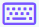Easy
Medium
Hard

## Double integral calculator with steps

Double integral calculator is used to solve the antiderivative of two-variable functions. This double integration calculator finds 2-dimensional integration with steps.
It can calculate the problems of the double definite integral as well as a double indefinite integral.

## How does the Double integration Calculator work?

Second integral calculator is an easy-to-use tool. Follow the below steps to calculate the second integral.

• Select the option first, definite or indefinite.
• Enter the two-variable function into the input box.
• Use the keypad iconto enter mathematical symbols.
• Enter the upper and lower limits of both the variables, in the case of the definite integral.
• Select the order of variables i.e., dxdy or dydx.
• Press the calculate key to get the result.
• Hit the reset key to enter a new function.

## What is double integral?

A double integral is a type of integral having two-variable function f(x, y) used to find the volume between the graph and a rectangular region of the xy-plane by taking an integral of an integral. The process of finding the double integral is known as double integration.

### Types:

• Definite integrals
• Indefinite integrals

The equation of double definite integral:

$$\int _{y_1}^{y_2}\left(\int _{x_1}^{x_2}f\left(x,y\right)dx\right)dy$$

The equation can also be written in other way if you want to calculate the second variable first.

$$\int _{x_1}^{x_2}\left(\int _{y_1}^{y_2}f\left(x,y\right)dy\right)dx$$

But in case of indefinite integral, the upper and lower limits are not used.

$$\int\int f\left(x,y\right)dxdy$$

In these equations

• f(x, y) is the double variable function.
• $$x_1,\:x_2,\:y_1,\:and\:y_2$$ are the upper and lower limits of double variable functions.
• And dx & dy are the variables of integration.

## How to calculate the double integrals?

Following are a few examples of double integrals solved by our double integrals calculator.

Example 1: For the definite integral

Find the double integral of $$x^2+2y^2$$ w.r.t x & y having limits from 1 to 3 for x and 2 to 4 for y?

Solution

Step 1: Write the given function along with the double integral notation.

$$\int _2^4\int _1^3\left(x^2+2y^2\right) dxdy$$

Step 2: Integrate the above expression w.r.t "x".

$$\int _2^4\left(\int _1^3\left(x^2+2y^2\right)dx\right)dy$$

$$\int _2^4\left(\int _1^3x^2dx+\int _1^3 2y^2dx\right)dy$$

$$\int _2^4\left(\left[\frac{x^{2+1}}{2+1}\right]^3_1+\left[2y^2x\right]^3_1\right)dy$$

$$\int _2^4\left(\frac{1}{3}\left[x^3\right]^3_1+2y^2\left[x\right]^3_1\right)dy$$

Apply the Fundamental theorem of calculus.

$$\int _2^4\left(\frac{1}{3}\left[3^3-1^3\right]+2y^2\left[3-1\right]\right)dy$$

$$\int _2^4\left(\frac{1}{3}\left[27-1\right]+2y^2\left[3-1\right]\right)dy$$

$$\int _2^4\left(\frac{26}{3}+4y^2\right)dy$$

Step 3: Now integrate w.r.t "y".

$$\int _2^4\frac{26}{3}dy+\int _2^44y^2dy$$

$$\left[\frac{26}{3}y\right]^4_2+\left[4\frac{y^{2+1}}{2+1}\right]^4_2$$

$$\frac{26}{3}\left[y\right]^4_2+\frac{4}{3}\left[y^3\right]^4_2$$

$$\frac{26}{3}\left[4-1\right]+\frac{4}{3}\left[4^3-2^3\right]$$

$$\frac{26}{3}\left[3\right]+\frac{4}{3}\left[64-8\right]$$

$$\left(26+\frac{4}{3}\left[56\right]\right)$$

$$26+\frac{224}{3}$$

$$\frac{302}{3}$$

$$100.67$$

Step 4: Now write the input with result.

$$\int _2^4\int _1^3\left(x^2+2y^2\right)dxdy=100.67$$

Example 2: For indefinite integral.

Find the double integral of xy with respect to x & y

Solution

Step 1: Write the given function along with the double integral notation.

$$\int \int xy\ dxdy$$

Step 2: Integrate the above expression w.r.t "x".

$$\int \left(\int {xy}dx\right)dy$$

$$\int \left(y\int x dx\right)dy$$

$$\int \left(y\left(\frac{x^2}{2}\right)+c_1\right)dy$$

$$\int \left(\frac{x^2\text{y}}{2}+c_1\right)dy$$

Step 3: Now integrate w.r.t "y".

$$\frac{x^2}{2}\int \text{y dy}+\int c_1dy$$

$$\frac{x^2}{2}\left(\frac{y^2}{2}\right)+c_1y+c_2$$

$$\frac{x^2y^2}{4}+c_1y+c_2$$

Step 4: Now write the input with result.

$$\int \int xy dxdy=\frac{x^2y^2}{4}+c_1y+c_2$$

## Resources

Use android or iOS app of our limit calculator on your mobile